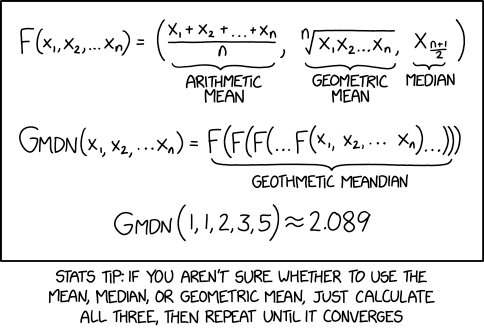## exxon prediction [xkcd]

Posted in Statistics with tags , , , , on September 7, 2021 by xi'an

## analyse des données

Posted in Books, Kids with tags , , , , , on June 24, 2021 by xi'an

## meandering

Posted in Books, Kids, R, Statistics with tags , , , , , , , on March 12, 2021 by xi'anA bit of a misunderstanding from Randall Munroe and then some: the function F returns a triplet, hence G should return a triplet as well. Even if the limit does return three identical values. And he should have also included the (infamous) harmonic mean! And the subtext (behind the picture) mentions random forest statistics, using every mean one can think of and dropping those that are doing worse, while here all solutions return the same value, hence do not directly discriminate between the averages (and there is no objective function to create the nodes in the trees, &tc.).

Here is a test R code including the harmonic mean:

```xkcd=function(x)c(mean(x),exp(mean(log(x))),median(x),1/mean(1/x))
xxxkcd=function(x,N=10)ifelse(rep(N==1,4),xkcd(x),xxxkcd(xkcd(x),N-1))
xxxkcd(rexp(11))
 1.018197 1.018197 1.018197 1.018197
```

## use data that does not need statistics

Posted in Books, Kids, Statistics with tags , , , , on March 2, 2021 by xi'an

## missing dimensions

Posted in Statistics with tags , , , , , , , , , , , , , on February 14, 2021 by xi'an